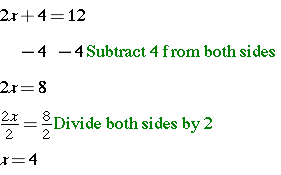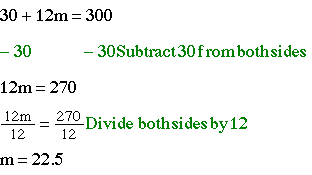# Solve Two-Step EquationsIn earlier lessons, you learned to solve one-step equations involving integers, decimals, and fractions. Now you will learn to solve two-step equations. In these types of equations, it is better to perform addition and subtraction before multiplication and division.   In other words, you still use inverse operations to solve these types of equations, but for these equations, you will reverse the order of operations when solving them. This rule remains in effect for any equation involving more than one operation. The ultimate goal in solving a two-step equation is the same as the goal of solving a one-step equation: to isolate the variable on one side of the equation.

Let’s get started!

### Solve two-step equations using DIVISION:

Example 12(4) + 4 = 12, 8 + 4 = 12

Example 2### Solve two-step equations using MULTIPLICATION:

Example## InvestigateA new one-year membership at Silver Fitness Center costs \$300. \$30 of the \$300 is paid up front as a registration fee and the remaining membership cost is paid monthly. How much do new members pay each month?

Strategy

Summarize the problem, using only key words or phrases.

The registration fee is a one-time payment and the rest of the \$300 will be divided over 12 months.

Registration fee plus 12 monthly payments is \$300. (Let m represent the monthly cost.)

So \$30 + 12m = \$300.00Interpret the solution:   It will cost \$22.50 per month for a one year membership.

## Solve Two-Step Equations Practice

Which equation in each pair has the larger solution? Write both answers with a greater than/less than symbol between them.

1.  a.  -1 – 3x = 8   or   b. -x + 3 = 3

2.  a.  3x – 1 = -13   or   b. 2 – 2r = 8

3.  a.  2v + 3 = 5   or   b. -3x – 2 = -12

(source)